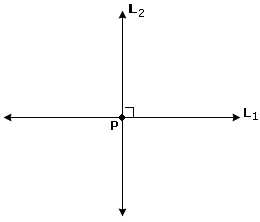## Definition Of Parallel And Perpendicular Lines

Parallel Lines are distinct lines lying in the same plane and they never intersect each other. Parallel lines have the same slope. In the figure below, lines PQ and RS are parallel and the lines l and m are parallel.Perpendicular lines are lines that intersect at right angles. If two lines are perpendicular to each other, then the product of their slopes is equal to - 1. In the figure shown below, the lines AB and EF are perpendicular to each other.### Solved Example on Parallel and Perpendicular Lines

#### Ques: Find the number of points of intersection for one pair of perpendicular lines.

##### Choices:

A. 3
B. 0
C. 2
D. 1